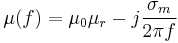# Material Property

The material property is used for materials that are simply defined by the constant permittivity εr, magnetic permeability μr and electric conductivity κ.

This material can be described as:$\varepsilon(f) = \varepsilon_0\varepsilon_r - j\frac{\kappa}{2\pi f}$
and$\mu(f) = \mu_0\mu_r - j\frac{\sigma_m}{2\pi f}$ ,where σm is the non-physical magnetic conductivity.

More complex, dispersive Materials can be defined using the Drude/Lorentz material model.

## Description

A material property is added by

CSX = AddMaterial(CSX, name)


with the following parameters:

• CSX: The original CSX structure
• name: name of the assigned property, must be unique

### Material Parameter Definition

The material parameters (e.g. permittivity, conductivity etc.) are defined using SetMaterialProperty:

CSX = SetMaterialProperty(CSX, name, varargin)


with the following parameters:

• CSX: The original CSX structure
• name: name of the material property (see above)

and the optional key/value parameter:

• Epsilon: relative electric permittivity (must be >=1)
• Mue: relative magnetic permeability (must be >=1)
• Kappa: electric conductivity (must be >=0)
• Sigma: magnetic conductivity (non-physical property, must be >=0)

## Example

Add a non-magnetic material with ε = 10.2:

CSX = AddMaterial(CSX,'MaterialName');
CSX = SetMaterialProperty( CSX, 'MaterialName', 'Epsilon', 10.2, 'Mue', 1 );